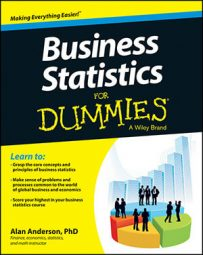In statistics, hypothesis testing refers to the process of choosing between competing hypotheses about a probability distribution, based on observed data from the distribution. It's a core topic and a fundamental part of the language of statistics.

Hypothesis testing is a six-step procedure:

1. Null hypothesis

2. Alternative hypothesis

3. Level of significance

4. Test statistic

5. Critical value(s)

6. Decision rule

The null hypothesis is a statement that's assumed to be true unless there's strong contradictory evidence. The alternative hypothesis is a statement that will be accepted in place of the null hypothesis if it is rejected.

The level of significance is chosen to control the probability of a "Type I" error; this is the error that results when the null hypothesis is erroneously rejected.

The test statistic and critical values are used to determine if the null hypothesis should be rejected. The decision rule that is followed is that an "extreme" test statistic results in rejection of the null hypothesis. Here, an extreme test statistic is one that lies outside the bounds of the critical value or values.

Hypotheses are often tested about the values of population measures such as the mean and the variance. They are also used to determine if a population follows a specified probability distribution. They also form a major part of regression analysis, where hypotheses are used to validate the results of an estimated regression equation.